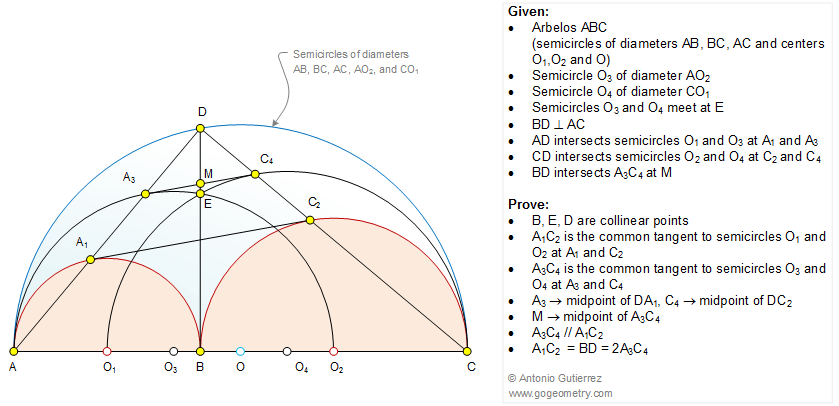Geometry Problem 1071: Arbelos, Semicircles, Diameter, Circle, Perpendicular, Common Tangent, Parallel, Collinear Points, Midpoint, 90 Degrees. Level: High School, College, Mathematics Education

< PREVIOUS PROBLEM  |  NEXT PROBLEM >

 The figure shows an arbelos ABC (AB, BC, and AC are semicircles of centers O1, O2, and O). The semicircles of diameters AO2 (center O3) and CO1 (center O4)meet at E. BD is perpendicular to AC, AD intersects semicircles O1 and O3 at A1 and A3, respectively, CD intersects semicircles O2 and O4 at C2 and C4, respectively. Prove that (1) B, E, D are collinear points; (2) A1C2 is the common tangent to semicircles O1 and O2 at A1 and C2, respectively; (3) A3C4 is the common tangent to semicircles O3 and O4 at A3 and C4, respectively; (4) A3 is the midpoint of DA1 and C4 is the midpoint of DC2; (5) M is the midpoint of A3C4; (6) A3C4 // A1A2; (7) A1C2 = BD = 2A3C4. See also: Artwork Problem 1071.Home | Search | Geometry | Problems | All Problems | Open Problems | Visual Index | 10 Problems | 1071-1080 | Arbelos | Semicircle | Circle | Tangent Circles | Circle Tangent Line | Diameter and Chord | Parallel lines | Perpendicular lines | Midpoint | Email | Solution / comment | by Antonio Gutierrez Last updated: Dec 28, 2014